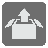Easy Unpack
Elementary
FR ZH-HANS JA English RU

Your mission here is to create a function that gets a tuple and returns a tuple with 3 elements - the first, third and second element from the last for the given array.One important thing worth pointing out is that you need to use index in order to extract elements from the array. Pay attention, index counting starts from 0, not from 1. Which means that if you need to get the first element from the array elements, you should do elements, and the second element is elements.

Input: A tuple, at least 3 elements long.

Output: A tuple.

Example:

```easy_unpack((6, 2, 9, 4, 3, 9)) == (6, 9, 3)
easy_unpack((1, 2, 3, 4, 5, 6, 7, 9)) == (1, 3, 7)
easy_unpack((1, 1, 1, 1)) == (1, 1, 1)
easy_unpack((6, 3, 7)) == (6, 7, 3)
```